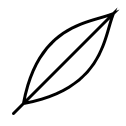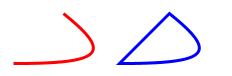# Paths

Paths are a way to construct complex shapes. A path specifies how to control a virtual pen to draw a line. There are three different commands that a path can contain:

• straight lines;
• bezier curves; and
• straight line movement that doesn't draw to the screen.

Here's an example of creating a path. Notice that it has examples of all of the different components above.

``````import doodle.core._
import doodle.syntax.all._

val path =
ClosedPath.empty
.lineTo(100, 100)
.curveTo(90, 75, 90, 25, 10, 10)
.moveTo(100, 100)
.curveTo(75, 90, 25, 90, 10, 10)``````

Drawing this path creates the output below.You probably noticed this is a `ClosedPath`, which suggests there is also an `OpenPath`. This is correct. The difference is how the path ends. If a `ClosedPath` doesn't end at the point it started (which is the origin), it will have a straight line inserted joining the end to the start. An `OpenPath` will not.

Here's an example showing the difference. We create a curve, drawn as an open and a closed path. Notice how the open path is just a curve, while the closed path has an extra line added joining the start and end points.

``````val open =
OpenPath.empty.curveTo(90, 0, 100, 10, 50, 50).path.strokeColor(Color.red)

val closed =
ClosedPath.empty.curveTo(90, 0, 100, 10, 50, 50).path.strokeColor(Color.blue)

val paths = open.beside(closed)``````Styling→